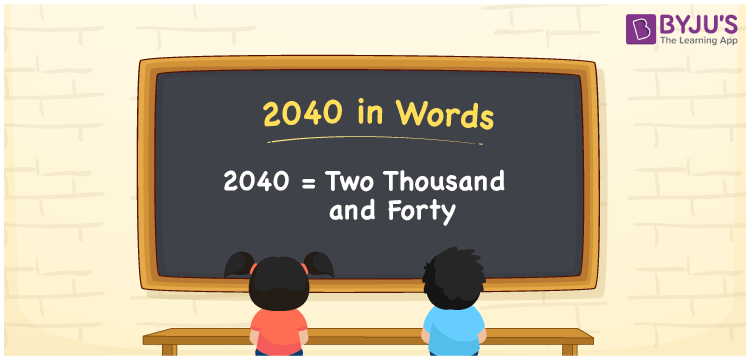# 2040 in Words

The number 2040 in English words is represented by “Two Thousand and Forty”. Students should learn the number names as it helps them to count the objects. The conversion from numbers to words is usually done with the help of the place value system. For example, if you saved Rs. 2040 in 3 months, you can write it as “I saved Rs. Two Thousand and Forty in three months”.

 2040 in Words: Two Thousand and Forty. Two Thousand and Forty in Numerical Form: 2040.

## 2040 in English Words## How to Write 2040 in Words?

The following table represents the place values of the four-digit number 2040. Go through the below table, and understand the procedure of writing 2040 in words.

 Thousands Hundreds Tens Ones 2 0 4 0

The expanded form of 2040 is as follows:

= 2 × Thousand + 0 × Hundred + 4 × Ten + 0 × One

= 2 × 1000 + 4 × 10

= 2000 + 40

= 2040

= two thousand and forty

Hence, 2040 in words is two thousand and forty.

2040 in words – Two thousand and forty

Is 2040 an odd number? – No

Is 2040 an even number? – Yes

Is 2040 a perfect square number? – No

Is 2040 a perfect cube number? – No

Is 2040 a prime number? – No

Is 2040 a composite number? – Yes

## Frequently Asked Questions on 2040 in Words

Q1

### How to write 2040 in words?

2040 in words is two thousand and forty.

Q2

### Simplify 2000 + 40, and express it in words.

Simplifying 2000 + 40, we get 2040. Hence, 2040 in words is two thousand and forty.

Q3

### Is 2040 a composite number?

Yes, 2040 is a composite number.